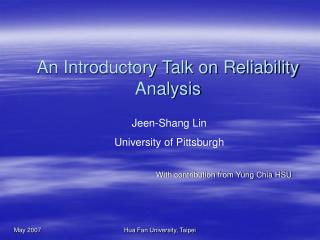# An Introductory Talk on Reliability Analysis - PowerPoint PPT PresentationDownload PresentationAn Introductory Talk on Reliability Analysis

An Introductory Talk on Reliability AnalysisDownload Presentation## An Introductory Talk on Reliability Analysis

- - - - - - - - - - - - - - - - - - - - - - - - - - - E N D - - - - - - - - - - - - - - - - - - - - - - - - - - -
##### Presentation Transcript

1. An Introductory Talk on Reliability Analysis Jeen-Shang Lin University of Pittsburgh With contribution from Yung Chia HSU Hua Fan University, Taipei

2. Supply vs. Demand • Failure takes place when demand exceeds supply. • For an engineering system: • Available resistance is the supply, R • Load is the demand, Q • Margin of safety, M=R-Q • The reliability of a system can be defined as the probability that R>Q represented as:

3. Risk • The probability of failure, or risk • How to find the risk? • If we known the distribution of M; • or, the mean and variance of M; • then we can compute P(M<0) easily.

4. Normal distribution: the bell curve For a wide variety of conditions, the distribution of the sum of a large number of random variables converge to Normal distribution. (Central Limit Theorem)

5. IF M=Q-R is normal When Because of symmetry Define reliability index

6. Example: vertical cut in clay If all variables are normal,

7. Some basics Negative coefficient

8. Engineers like Factor of safety • F=R/Q, if F is normal reliability index

9. Lognormal distribution • The uncertain variable can increase without limits but cannot fall below zero. • The uncertain variable is positively skewed, with most of the values near the lower limit. • The natural logarithm of the uncertain variable follows a normal distribution. F is also often treated as lognormal

10. In case of lognormal Ln(R) and ln(Q) each is normal

11. First order second moment method • The MFOSM method assumes that the uncertainty features of a random variable can be represented by its first two moments: mean and variance. • This method is based on the Taylor series expansion of the performance function linearized at the mean values of the random variables.

12. First order second moment method • Taylor series expansion

13. Example: vertical cut in clay If all variables are normal, 1-normcdf(1.8896,0,1) MATLAB

14. Slope stability 2 (H): 1(V) slope with a height of 5m

15. Reliability Analysis • The reliability of a system can be defined as the probability that R>Q represented as:

16. FS contour , , 0.21.

17. First Order Reliability Method Hasofer-Lind (FORM) • Probability of failure can be found obtained in material space • Approximate as distance to Limit state

18. Distance to failure criterion • If F=1 or M=0 is a straight line • Reliability becomes the shortest distance

19. Constraint Optimization:Excel

20. May get similar results with FOSM FOSM 1-normcdf(1. 796,0,1)=0.0362 MATLAB

21. Monte Carlo Simulationcorrelation=0 Monte Carlo=0.0495

22. Monte Carlo Simulationcorrelation=0.5 FORM=0.0362

23. The matrix form of the Hasofer-Lind (1974) FS=1.0 (M=0) UNSAFE Region FS<1 or M<0

24. Soil properties FOS=1 Soil properties>0 The matrix form of the Hasofer-Lind (1974)

25. FS=1.0 Correlation=.99 UNSAFE Region FS<1 or M<0 Correlation=-.99 Correlation=0

26. The distance

27. FOSM maybe wrong • FOSM

28. A projection Method • Check the FOSM • Use the slope, projected to where the failure material is • Use the material to find FS • If FS=1, ok

29. Hua Fan University, Taipei